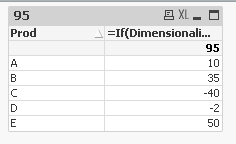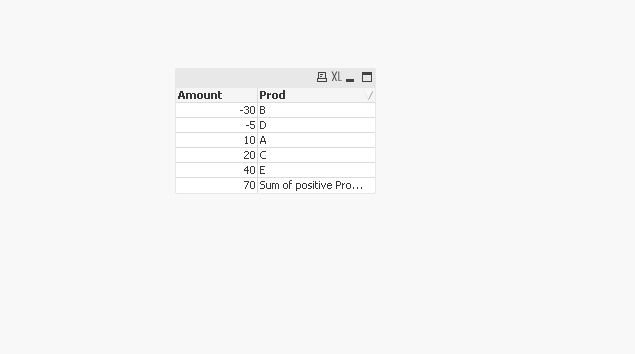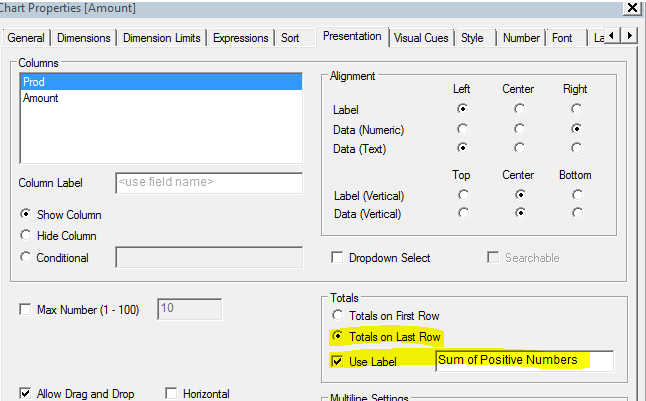# New to QlikView

Discussion board where members can get started with QlikView.

Contributor II

## Need logic help

I attached file,

I want to show only the sum of Positive numbers. and in table all number should be present (Positive & Negative).

Thanks,

Lalit kumar

1 Solution

Accepted SolutionsMVP

## Re: Need logic help

If in case you have more than one amount value per Prod and you want to check if the Sum(Amount) is greater than 0, then try this

=If(Dimensionality() = 0,Sum({<Prod = {"=Sum(Amount) > 0"}>}Amount), Sum(Amount))7 RepliesPartner

## Re: Need logic help

Hi lalit,

try this expression, it will show only positive values..

=if(sum(Amount)>=0,sum(Amount))

Valued Contributor

## Re: Need logic help

hi,

Please check the attached QVW.

Please mark the question as answered if it meets ur requirement .!Partner

## Re: Need logic help

Try this.

Use this expression:

=sum(if(Amount>0,Amount))

I hope this might hekps you

-NagarjunMVP

## Re: Need logic help

I don't have license, Can you please check for this on your application whether this is working or not

=sum({<Amount = {'>0'}>} Amount * 1)

Before develop something, think If placed (The Right information | To the right people | At the Right time | In the Right place | With the Right context)
Honored Contributor II

## Re: Need logic help

try like this:

=if(sum(Amount)>0,sum(Amount),text(sum(Amount)))

Highlighted
Contributor III

## Re: Need logic help

Hi

Please find the attached app.

Expression:

if( Dimensionality()=0 , sum({<Amount={">0"}>}Amount) , Sum(Amount) )

Few changes in the Presentation Tab,Hope this helps!

Regards

HarishMVP

## Re: Need logic help

If in case you have more than one amount value per Prod and you want to check if the Sum(Amount) is greater than 0, then try this

=If(Dimensionality() = 0,Sum({<Prod = {"=Sum(Amount) > 0"}>}Amount), Sum(Amount))